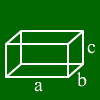# Cuboid calculation

For cuboids those formulas hold:
base area = a*b
volume = a*b*c
surface=2*a*b + 2*a*c + 2*b*c

## What is a cuboid?

A cuboid is a solid where all edges are perpendicular on each other. Therefore, a cuboid has rectangles as faces. So we can see there are always 4 edges having the same length.

## How to do cuboid calculations?

Most calculations are very simple and directly be seen from rectangle formulas. For example the surface consists of six rectangles, pairs of two of them having the same area. So the formula is 2*a*b + 2*b*c + 2*a*c.
The diagonal from one corner to the opposite corner has the formula square root of (a²+b²+c²) as we can see by applying Pythagoras twice.
A cuboid is uniquely determined by three of its lengths.

If you want to see some examples, just enter your values above.

For further information, just move the mouse over one of the words below and the corresponding piece of the cuboid gets marked.Side a
Side b
Side c
diagonal
surface
volume

## Cuboid calculation

Here you got a free cuboid calculator. Just enter edges, height, volume or diagonal.